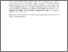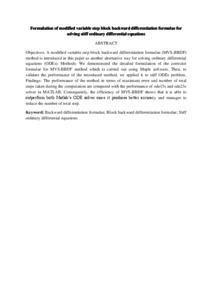Simple Search:

Formulation of modified variable step block backward differentiation formulae for solving stiff ordinary differential equations

Citation

Asnor, Asma Izzati and Mohd Yatim, Siti Ainor and Ibrahim, Zarina Bibi (2017) Formulation of modified variable step block backward differentiation formulae for solving stiff ordinary differential equations. Indian Journal of Science and Technology, 10 (12). p. 43106. ISSN 0974-6846; ESSN: 0974-5645

Abstract / Synopsis

Objectives: A modified variable step block backward differentiation formulae (MVS-BBDF) method is introduced in this paper as another alternative way for solving ordinary differential equations (ODEs). Methods: We demonstrated the detailed formulation of the corrector formulae for MVS-BBDF method which is carried out using Maple software. Then, to validate the performance of the introduced method, we applied it to stiff ODEs problem. Findings: The performance of the method in terms of maximum error and number of total steps taken during the computation are compared with the performance of ode15s and ode23s solver in MATLAB. Consequently, the efficiency of MVS-BBDF shows that it is able to outperform both Matlab’s ODE solver since it produces better accuracy and manages to reduce the number of total step.Preview
PDF
Formulation of modified variable step block backward differentiation formulae for solving stiff ordinary differential equations .pdfView Item